Open in App
Not now

# SQL – count() with Group By clause

• Last Updated : 25 Oct, 2021

The count() function is an aggregate function use to find the count of the rows that satisfy the fixed conditions. The count() function with the GROUP BY clause is used to count the data which were grouped on a particular attribute of the table.

Syntax:

```SELECT attribute1 , COUNT(attribute2)
FROM table_name
GROUP BY attribute1```

In this article let us see the SQL count() with Group by clause using MSSQL as a server.

Step 1: We are creating a Database. For this use the below command to create a database named GeeksforGeeks.

Query:

`CREATE DATABASE GeeksforGeeks;`Step 2: To use the GeeksforGeeks database use the below command.

Query:

`USE GeeksforGeeks`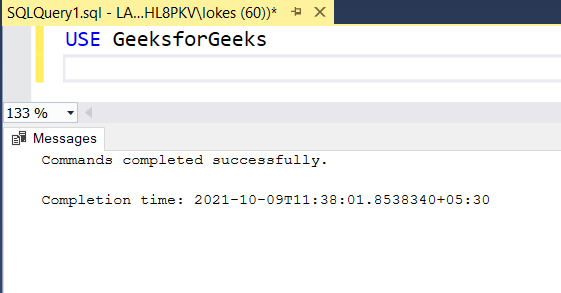Step 3: Now we are creating a table. Create a table student_marks with 4 columns using the following SQL query.

Query:

```CREATE TABLE student_marks(
stu_id VARCHAR(20),
stu_name VARCHAR(20),
stu_branch VARCHAR(20),
total_marks INT
)```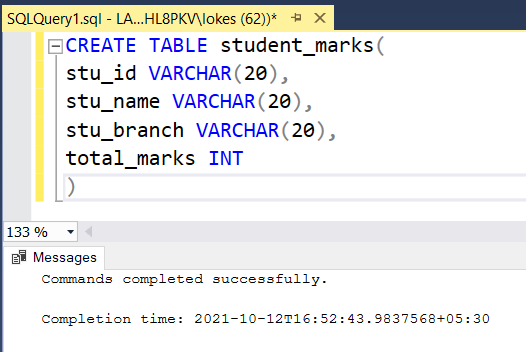Step 4: Viewing the description of the table.

Query:

`EXEC sp_columns student_marks`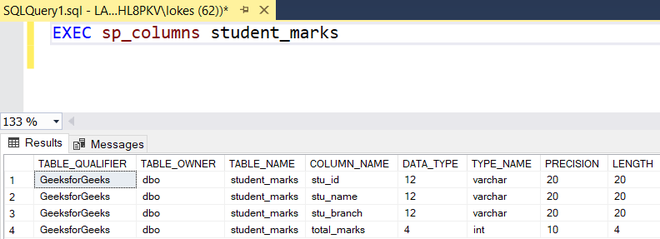Step 5: The query for Inserting rows into the Table. Inserting rows into student_marks  table using the following SQL query.

Query:

```INSERT INTO student_marks
( '1002','KIRAN','E.C.E', 540),
( '1003','PRANAV','E.C.E', 450),
( '2002','SRUTHI','C.S.E', 480),
( '2003','HARSITHA','C.S.E', 534),
( '3001','SAI','I.T', 560),
( '3002','HARSH','I.T', 510),
( '3003','HARSHINI','I.T', 500)```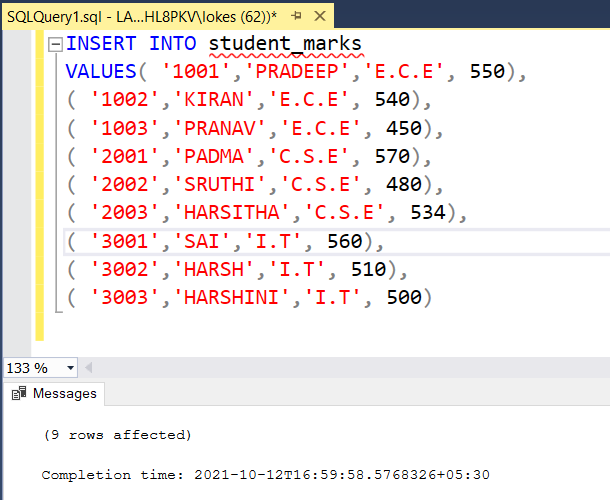Step 6: Viewing the inserted data

Query:

`SELECT * FROM student_marks`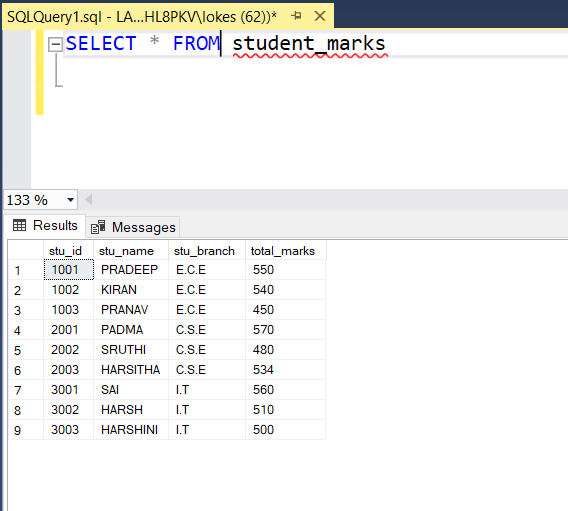• Query to find the number of students in each branch
```SELECT stu_branch, COUNT(stu_id) AS number_of_students
FROM student_marks
GROUP BY stu_branch```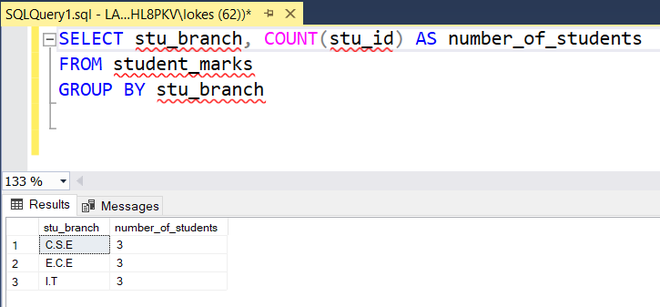• Query to find the number of students whose marks are greater than the average marks of the table.
```SELECT AVG(total_marks) AS average,COUNT(stu_id) AS number_of_students
FROM student_marks
WHERE total_marks>(SELECT AVG(total_marks) FROM student_marks)```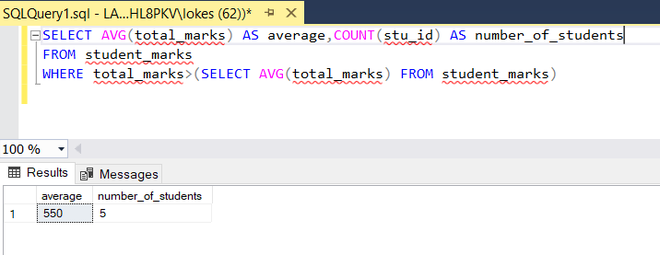My Personal Notes arrow_drop_up